# RD Sharma Solutions for Class 8 Maths Chapter 5 - Playing with Numbers Exercise 5.3

Students can refer and download RD Sharma Class 8 Maths Chapter 5 Playing with Numbers from the links provided below. Subject experts have solved the RD Sharma Class 8 Solutions to help students grasp the concepts easily, and also helps in boosting their confidence level, which plays an essential role in their examinations. Hence, students whose intention is to score high in the exams are advised to go through RD Sharma Class 8 Solutions.

In Exercise 5.3 we shall discuss problems based on addition and multiplication of cryptarithms, (cryptarithms are puzzles, which are operated on various operations and numbers, in which letters take the place of digits and one has to find out which letter represents which digit).

## Download the pdf of RD Sharma Solutions For Class 8 Maths Chapter 5 Playing with Numbers Exercise 5.3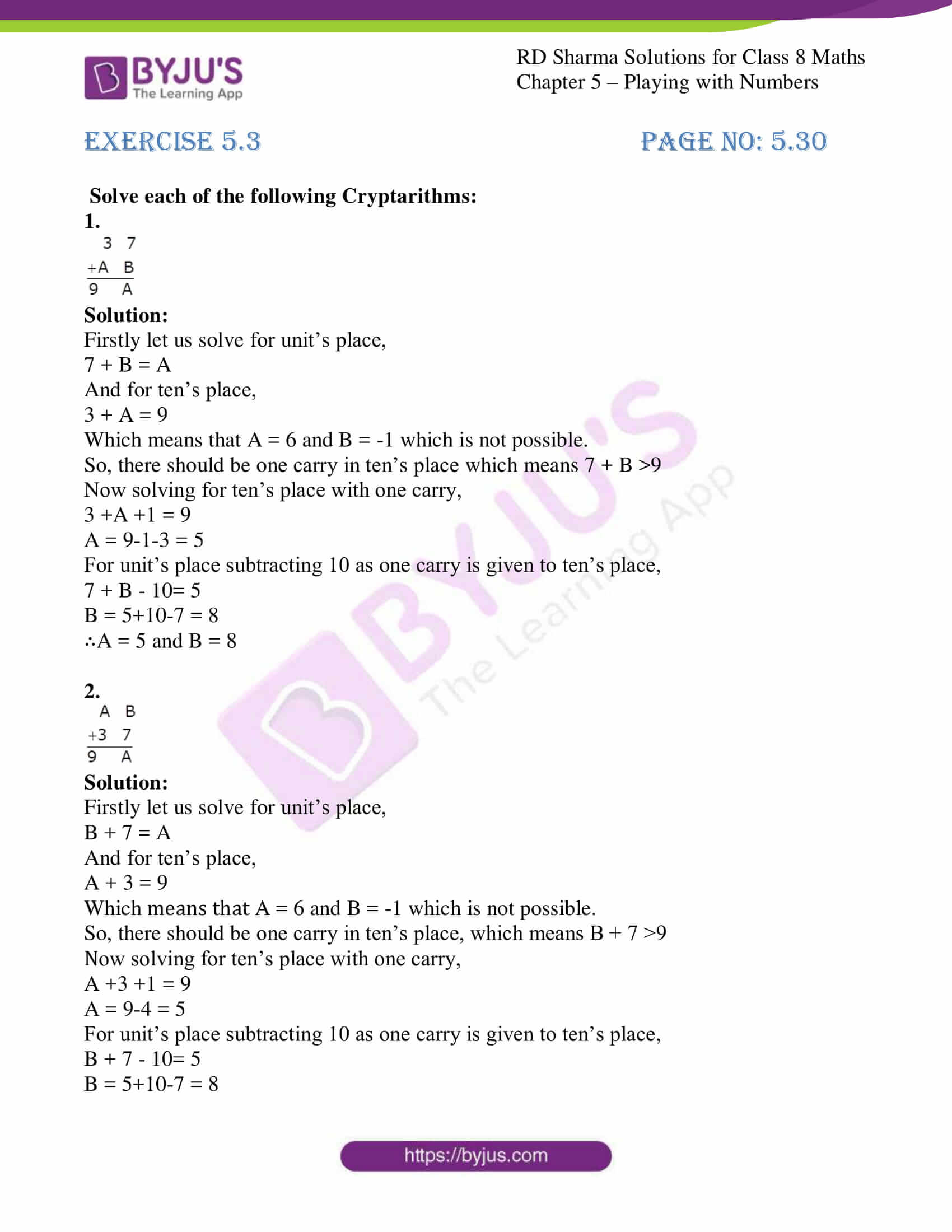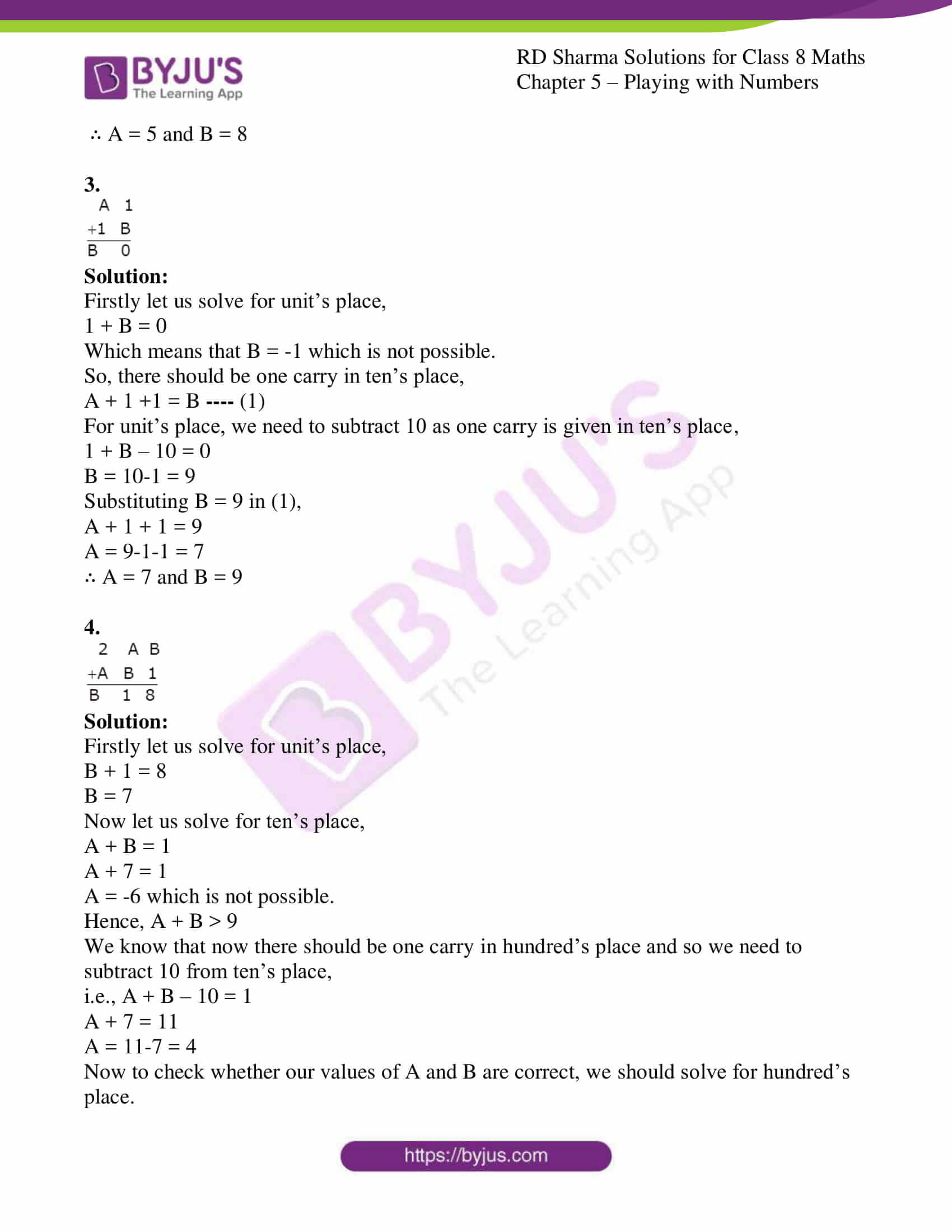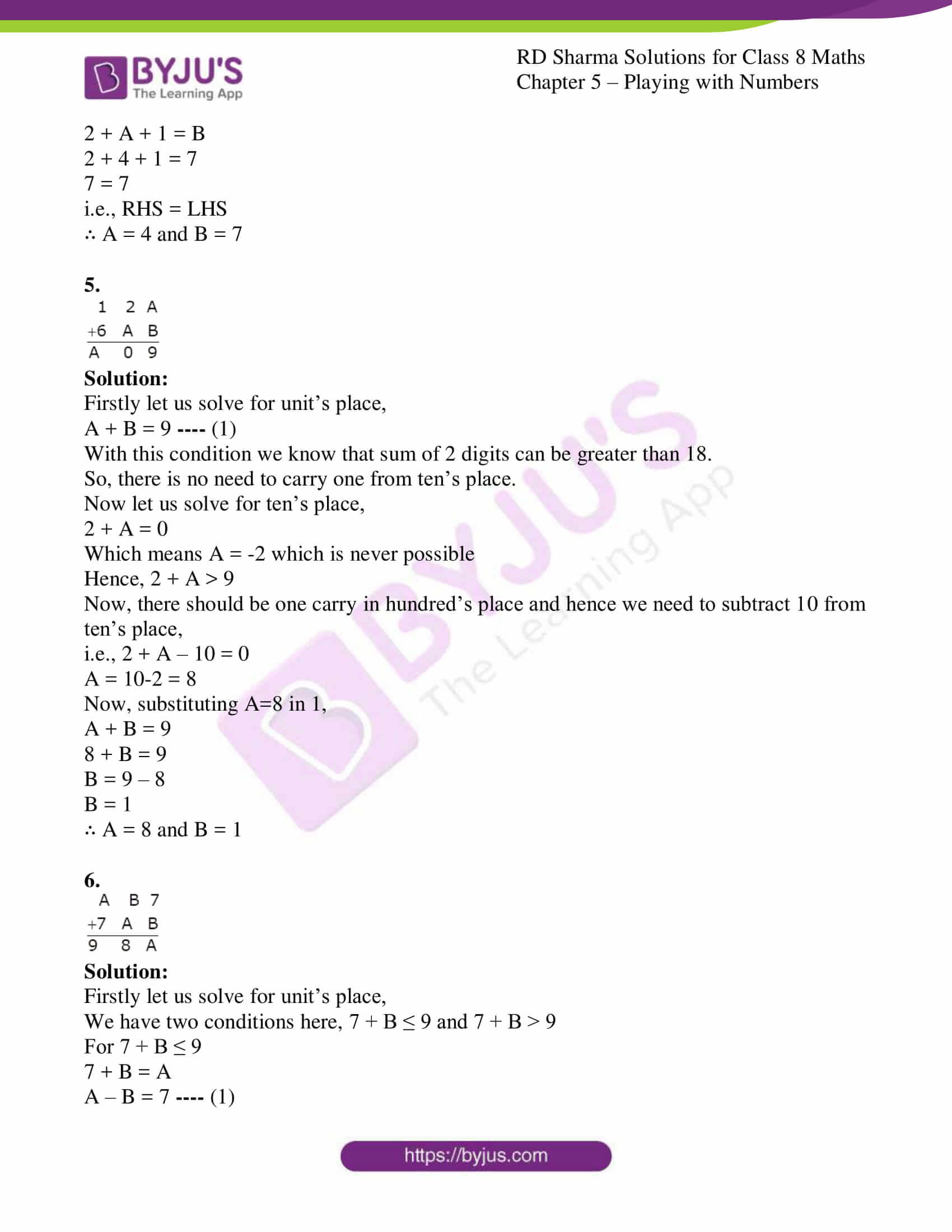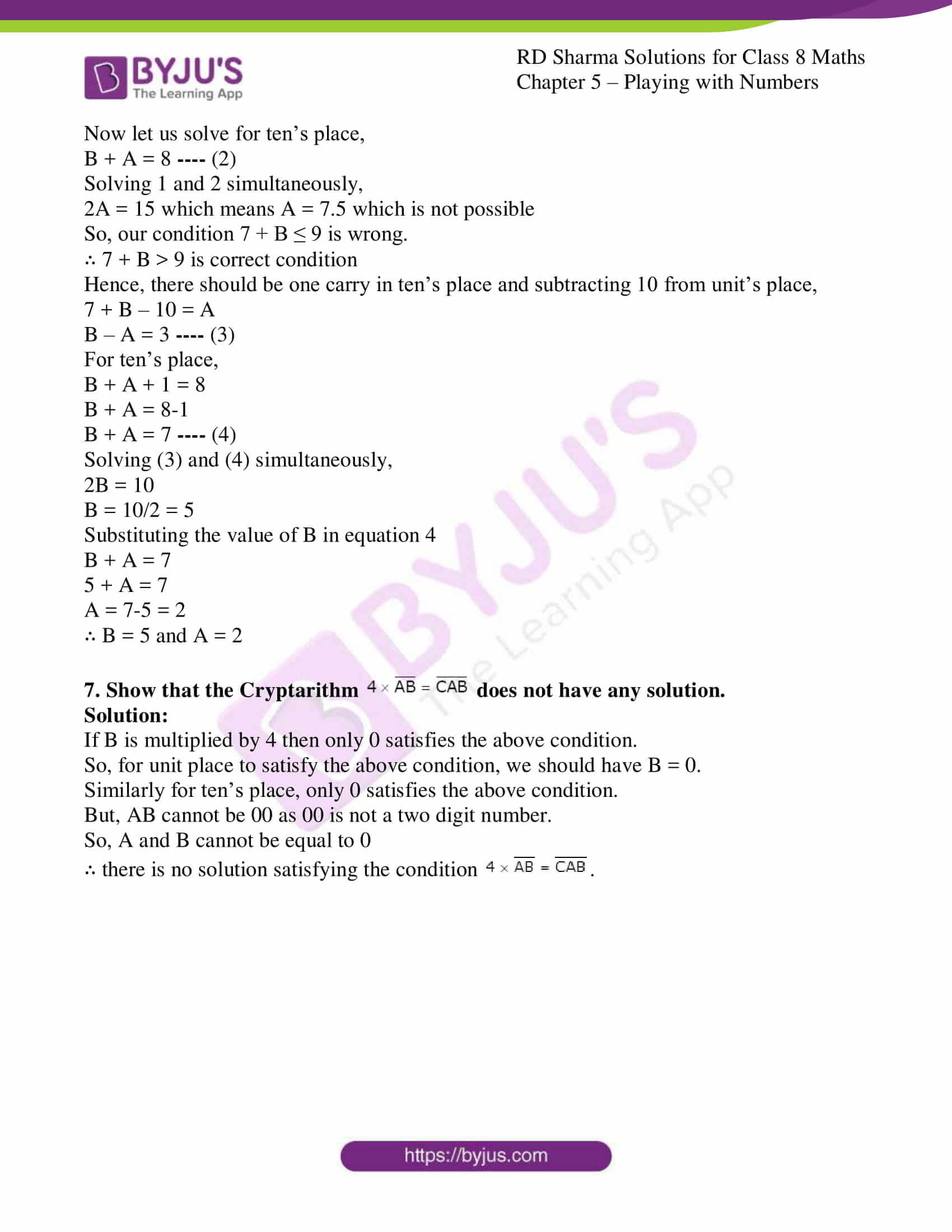### Access answers to Maths RD Sharma Solutions For Class 8 Exercise 5.3 Chapter 5 – Playing with Numbers

Solve each of the following Cryptarithms:
1.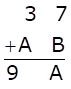Solution:

Firstly let us solve for unit’s place,

7 + B = A

And for ten’s place,

3 + A = 9

Which means that A = 6 and B = -1 which is not possible.

So, there should be one carry in ten’s place which means 7 + B >9

Now solving for ten’s place with one carry,

3 +A +1 = 9

A = 9-1-3 = 5

For unit’s place subtracting 10 as one carry is given to ten’s place,

7 + B – 10= 5

B = 5+10-7 = 8

∴A = 5 and B = 8

2.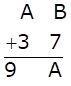Solution:

Firstly let us solve for unit’s place,

B + 7 = A

And for ten’s place,

A + 3 = 9

Which means that A = 6 and B = -1 which is not possible.

So, there should be one carry in ten’s place, which means B + 7 >9

Now solving for ten’s place with one carry,

A +3 +1 = 9

A = 9-4 = 5

For unit’s place subtracting 10 as one carry is given to ten’s place,

B + 7 – 10= 5

B = 5+10-7 = 8

∴ A = 5 and B = 8

3.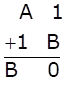Solution:

Firstly let us solve for unit’s place,

1 + B = 0

Which means that B = -1 which is not possible.

So, there should be one carry in ten’s place,

A + 1 +1 = B —- (1)

For unit’s place, we need to subtract 10 as one carry is given in ten’s place,

1 + B – 10 = 0

B = 10-1 = 9

Substituting B = 9 in (1),

A + 1 + 1 = 9

A = 9-1-1 = 7

∴ A = 7 and B = 9

4.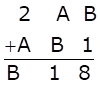Solution:

Firstly let us solve for unit’s place,

B + 1 = 8

B = 7

Now let us solve for ten’s place,

A + B = 1

A + 7 = 1

A = -6 which is not possible.

Hence, A + B > 9

We know that now there should be one carry in hundred’s place and so we need to subtract 10 from ten’s place,

i.e., A + B – 10 = 1

A + 7 = 11

A = 11-7 = 4

Now to check whether our values of A and B are correct, we should solve for hundred’s place.

2 + A + 1 = B

2 + 4 + 1 = 7

7 = 7

i.e., RHS = LHS

∴ A = 4 and B = 7

5.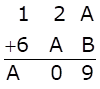Solution:

Firstly let us solve for unit’s place,

A + B = 9 —- (1)

With this condition we know that sum of 2 digits can be greater than 18.

So, there is no need to carry one from ten’s place.

Now let us solve for ten’s place,

2 + A = 0

Which means A = -2 which is never possible

Hence, 2 + A > 9

Now, there should be one carry in hundred’s place and hence we need to subtract 10 from ten’s place,

i.e., 2 + A – 10 = 0

A = 10-2 = 8

Now, substituting A=8 in 1,

A + B = 9

8 + B = 9

B = 9 – 8

B = 1

∴ A = 8 and B = 1

6.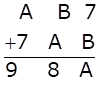Solution:

Firstly let us solve for unit’s place,

We have two conditions here, 7 + B ≤ 9 and 7 + B > 9

For 7 + B ≤ 9

7 + B = A

A – B = 7 —- (1)

Now let us solve for ten’s place,

B + A = 8 —- (2)

Solving 1 and 2 simultaneously,

2A = 15 which means A = 7.5 which is not possible

So, our condition 7 + B ≤ 9 is wrong.

∴ 7 + B > 9 is correct condition

Hence, there should be one carry in ten’s place and subtracting 10 from unit’s place,

7 + B – 10 = A

B – A = 3 —- (3)

For ten’s place,

B + A + 1 = 8

B + A = 8-1

B + A = 7 —- (4)

Solving (3) and (4) simultaneously,

2B = 10

B = 10/2 = 5

Substituting the value of B in equation 4

B + A = 7

5 + A = 7

A = 7-5 = 2

∴ B = 5 and A = 2

7. Show that the Cryptarithm does not have any solution.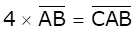Solution:

If B is multiplied by 4 then only 0 satisfies the above condition.

So, for unit place to satisfy the above condition, we should have B = 0.

Similarly for ten’s place, only 0 satisfies the above condition.

But, AB cannot be 00 as 00 is not a two digit number.

So, A and B cannot be equal to 0

∴ there is no solution satisfying the condition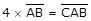.